##Circumconics of the triangle

There are many ways to define a circumconic of a triangle. The most prominent circumconic being the circumcircle, there is a natural way to obtain all others via projectivities applied to this prominent one. As explained in AllConicsCircumscribedTria.html , all these conics are uniquely defined by a point D and its trilinear polar. D defines namely a projectivity FD fixing the three vertices of the triangle and mapping the symmedian point K to D. The conic then is defined through the image c'=FD(c) of the circumcircle. FD maps the trilinear polar of K, which is the Lemoine axis of the triangle to the trilinear polar of the point D. In addition it maps the tangents to the circumcircle at the vertices of ABC to the corresponding tangents at the same vertices of c'.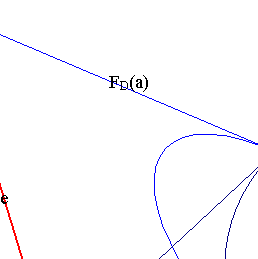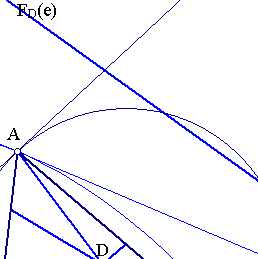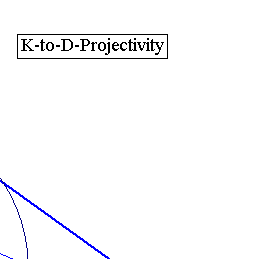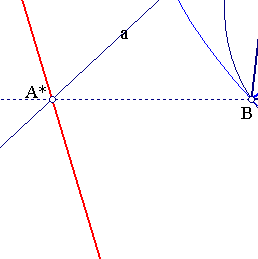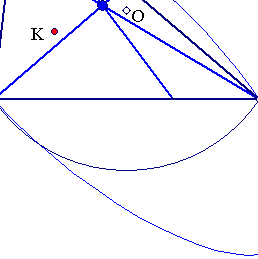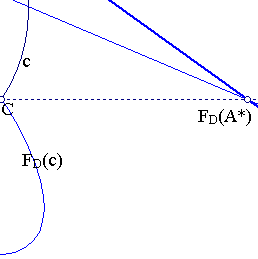Notice that instead of the symmedian point one could select some other arbitrary point K' and consider the projectivity fixing the vertices and sending K' to D. In that case each circumconic would be the image of the conic defined through the point K'. By selecting though K we arive at obtaining each circumconic as the image of the circumcircle under a very simple to define projectivity.
By selecting the K' to be the centroid G we get all circumconics as images of the outer Steiner ellipse of triangle ABC. The arguments at the end of ProjectivityFixingVertices.html show also the locations of D for which the corresponding conic is an ellipse, parabola or hyperbola. It is correspondingly the inner/on/outside of the inner Steiner ellipse of ABC.
A slightly different method to obtain all conics through one projectivity mapping the equilateral to an arbitrary triangle is considered in TriangleCircumconics2.html .